Open Access Publications from the University of California

## A combination theorem for Anosov subgroups

(2019)

We prove an analogue of Klein combination theorem for Anosov subgroups by using a local-to-global principle for Morse quasigeodesics.

• 1 supplemental PDF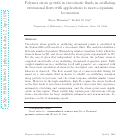## Polymer stress growth in viscoelastic fluids in oscillating extensional flows with applications to micro-organism locomotion

(2019)

Simulations of undulatory swimming in viscoelastic fluids with large amplitude gaits show concentration of polymer elastic stress at the tips of the swimmers. We use a series of related theoretical investigations to probe the origin of these concentrated stresses. First the polymer stress is computed analytically at a given oscillating extensional stagnation point in a viscoelastic fluid. The analysis identifies a Deborah number (De) dependent Weissenberg number (Wi) transition below which the stress is linear in Wi, and above which the stress grows exponentially in Wi. Next, stress and velocity are found from numerical simulations in an oscillating 4-roll mill geometry. The stress from these simulations is compared with the theoretical calculation of stress in the decoupled (given flow) case, and similar stress behavior is observed. The flow around tips of a time-reversible flexing filament in a viscoelastic fluid is shown to exhibit an oscillating extension along particle trajectories, and the stress response exhibits similar transitions. However in the high amplitude, high De regime the stress feedback on the flow leads to non time-reversible particle trajectories that experience asymmetric stretching and compression, and the stress grows more significantly in this regime. These results help explain past observations of large stress concentration for large amplitude swimmers and non-monotonic dependence on De of swimming speeds.

## Geometric finiteness in negatively pinched Hadamard manifolds

(2019)

In this paper, we generalize Bonahon's characterization of geometrically infinite torsion-free discrete subgroups of PSL(2,C) to geometrically infinite discrete subgroups Γ of isometries of negatively pinched Hadamard manifolds X. We then generalize a theorem of Bishop to prove that every discrete geometrically infinite isometry subgroup Γ has a set of nonconical limit points with the cardinality of the continuum.

• 1 supplemental PDF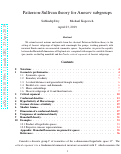## Patterson-Sullivan theory for Anosov subgroups

(2019)

We extend several notions and results from the classical Patterson-Sullivan theory to the setting of Anosov subgroups of higher rank semisimple Lie groups, working primarily with invariant Finsler metrics on associated symmetric spaces. In particular, we prove the equality between the Hausdorff dimensions of flag limit sets, computed with respect to a suitable Gromov (pre-)metric on the flag manifold, and the Finsler critical exponents of Anosov subgroups.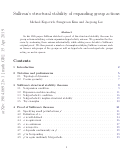## Structural stability of meandering-hyperbolic group actions

(2019)

In his 1985 paper Sullivan sketched a proof of his structural stability theorem for differentiable group actions satisfying certain expansion-hyperbolicity axioms. In this paper we relax Sullivan's axioms and introduce a notion of "meandering hyperbolicity" for group actions on geodesic metric spaces. This generalization is substantial enough to encompass actions of certain non-hyperbolic groups, such as actions of "uniform lattices" in semisimple Lie groups on flag manifolds. At the same time, our notion is sufficiently robust and we prove that meandering-hyperbolic actions are still structurally stable. We also prove some basic results on meandering-hyperbolic actions and give other examples of such actions.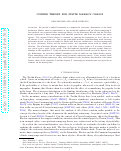## Unified theory for finite Markov chains

(2019)

We provide a unified framework to compute the stationary distribution of any finite irreducible Markov chain or equivalently of any irreducible random walk on a finite semigroup S. Our methods use geometric finite semigroup theory via the Karnofsky–Rhodes and the McCammond expansions of finite semigroups with specified generators; this does not involve any linear algebra. The original Tsetlin library is obtained by applying the expansions to P(n), the set of all subsets of an n element set. Our set-up generalizes previous groundbreaking work involving left-regular bands (or R-trivial bands) by Brown and Diaconis, extensions to R-trivial semigroups by Ayyer, Steinberg, Thiéry and the second author, and important recent work by Chung and Graham. The Karnofsky–Rhodes expansion of the right Cayley graph of S in terms of generators yields again a right Cayley graph. The McCammond expansion provides normal forms for elements in the expanded S. Using our previous results with Silva based on work by Berstel, Perrin, Reutenauer, we construct (infinite) semaphore codes on which we can define Markov chains. These semaphore codes can be lumped using geometric semigroup theory. Using normal forms and associated Kleene expressions, they yield formulas for the stationary distribution of the finite Markov chain of the expanded S and the original S. Analyzing the normal forms also provides an estimate on the mixing time.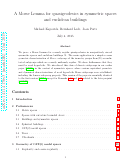## A Morse lemma for quasigeodesics in symmetric spaces and euclidean buildings

(2018)

We prove a Morse lemma for regular quasigeodesics in nonpositively curved symmetric spaces and euclidean buildings. We apply it to give a new coarse geometric characterization of Anosov subgroups of the isometry groups of such spaces simply as undistorted subgroups which are uniformly regular.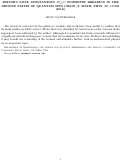## Editor’s Note: Spontaneous SU2( C ) symmetry breaking in the ground states of quantum spin chain [J. Math. Phys. 59, 111701 (2018)]

(2018)

The reviewers contacted by the editors to evaluate this work have been unable to confirm that the main results are correct. Flaws that were identified by the reviewers in earlier versions of the paper have been addressed by the author. Although it is possible that future research will uncover a significant mistake in this paper or show that the conclusions are in error, I believe that publishing it may benefit the readership of the Journal and stimulate further work in mathematical physics on an important topic.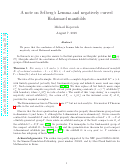## A note on Selberg's Lemma and negatively curved Hadamard manifolds

(2018)

Answering a question by Margulis we prove that the conclusion of Selberg's Lemma fails for discrete isometry groups of negatively curved Hadamard manifolds.